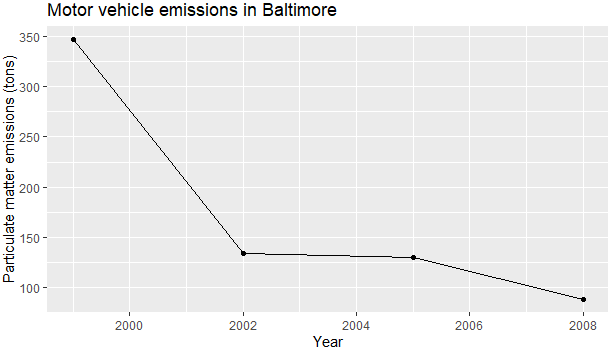2 views

With this data frame ("df"):

year   pollution

1 1999 346.82000

2 2002 134.30882

3 2005 130.43038

4 2008  88.27546

I try to create a line chart like this:

plot5 <- ggplot(df, aes(year, pollution)) +

geom_point() +

geom_line() +

labs(x = "Year", y = "Particulate matter emissions (tons)", title = "Motor vehicle emissions in Baltimore")

The error I get is:

geom_path: Each group consist of only one observation. Do you need to adjust the group aesthetic?

The chart appears as a scatter plot even though I want a line chart. I tried to replace geom_line() with geom_line(aes(group = year)) but that didn't work.

In an answer, I was told to convert the year to a factor variable. I did and the problem persists. This is the output of str(df) and dput(df):

'data.frame':   4 obs. of  2 variables:

\$ year     : num  1 2 3 4

\$ pollution: num [1:4(1d)] 346.8 134.3 130.4 88.3

..- attr(*, "dimnames")=List of 1

.. ..\$ : chr  "1999" "2002" "2005" "2008"

structure(list(year = c(1, 2, 3, 4), pollution = structure(c(346.82,

134.308821199349, 130.430379885892, 88.275457392443), .Dim = 4L, .Dimnames = list(

c("1999", "2002", "2005", "2008")))), .Names = c("year",

"pollution"), row.names = c(NA, -4L), class = "data.frame")

by

To remove this error, use any one of the following ways:

Convert factor columns to numeric:

In the data frame below the year column needs to be converted to a numeric to get rid of this error:

df <- data.frame(year = c("1999", "2002", "2005", "2008"),

pollution = c(346.82,134.308821199349, 130.430379885892, 88.275457392443))

str(df)

'data.frame': 4 obs. of  2 variables:

\$ year     : Factor w/ 4 levels "1999","2002",..: 1 2 3 4

\$ pollution: num  346.8 134.3 130.4 88.3

To convert to numeric:

df\$year = as.numeric(as.character(df\$year))

str(df)

'data.frame': 4 obs. of  2 variables:

\$ year     : num  1999 2002 2005 2008

\$ pollution: num  346.8 134.3 130.4 88.3

Plotting the values:

ggplot(df, aes(year, pollution)) +

geom_point() +

geom_line() +

labs(x = "Year", y = "Particulate matter emissions (tons)",title = "Motor vehicle emissions in Baltimore")

Output:You can also add group = 1 into the ggplot or geom_line aes() because for line graphs, the data points must be grouped so that it knows which points to connect.i.e.,

ggplot(df, aes(year, pollution, group = 1)) + geom_point() + geom_line() + labs(x = "Year", y = "Particulate matter emissions (tons)", title = "Motor vehicle emissions in Baltimore")